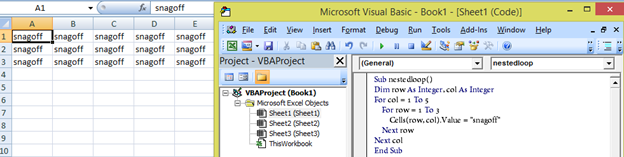### VBA MODULE 8

#### Program flow Control and Decisions with Loops

Looping means repetition. It is useful when we need to execute the same code numerous times until it meets an end condition.

In VBA we will find several control system which helps us to perform looping for complex programming.

In VBA, there are four types of loop

• The For Next Loop
• For Each Next Loop
• The Do While Loop
• The Do Until Loop

For Next Loop

Commonly used loop is For Next Loop. We always need to declare a variable to assign a range for our loop. The VBA code within the For Next statement is then executed for each value in the range.

To make it very clear let’s take an example to add numbers from 1 to 100 and display the total on the screen

 Sub adding() Dim Range as Integer Dim Total as Integer For Range =1 to 100 Total = Total +Range Next Range Msgbox Total End Sub

Explanation of the above example:

• We started with the Subroutine named “adding”
• Dim is used to declare variables Range and Total.
• In the For statement we declare a range for loop using Range variable
• Total variable will store the value every time the loop runs (till 100 times)
• Next will help to take the next value of Range
• After the loop executes 100 times it will end and Total will be displayed on the screen using msgbox

Nested Loop

Nested loop is loop within the loop.

Check the below example of nested For loop which will put same value to all the cells of the defined range

 Sub nestedloop() Dim row As Integer, col As Integer For col = 1 To 5     For row = 1 To 3         Cells(row, col).Value = “Snagoff”     Next row Next col End Sub

Result when you run the above program:The For Each Next Loop

The For Each Next loop is used when there is a need to loop through each object in a collection of objects. So instead of running through a set of values for a variable, it runs around the collection of objects.

Example:

This loops through each cell in the Range("A1:A5"), and the code sets font color of red in each cell. Here, Cellvalue is the Range Object variable, and the group or collection are all Cells in the Range("A1:A5").

 Sub fontcoloring() Dim Cellvalue As Range For Each Cellvalue In ActiveSheet.Range("A1:A5") Cellvalue.Font.Color = RGB(255, 0, 0) Next Cellvalue End Sub

Do While Loop

In Do While loop you don't need to set a start condition, just the end condition. It continues until a specified condition is met.

The following example uses a Do-While loop. This code uses the active cell as a starting point and then travels down the column, multiplying each cell’s value by 2 and showing the result in the next column using offset property. The loop continues until the routine encounters an empty cell.

 Sub DoWhileDemo() Do While ActiveCell.Value <> Empty  ActiveCell.Offset(0, 1).Value = ActiveCell.Value * 2  ActiveCell.Offset(1, 0).Select Loop End Sub

Do Until Loop

The Do-Until loop structure is similar to the Do-While structure. The two structures differ in their handling of the tested condition. A program continues to execute a Do-While loop while the condition remains true. In a Do-Until loop, the program executes the loop until the condition is true

Example

This code color the cells yellow, until a empty cell is encountered.

 Sub example() Dim rowNo As Integer rowNo = 1 Do Cells(rowNo, 1).Interior.Color = RGB(255, 255, 0) rowNo = rowNo + 1 Loop Until IsEmpty(Cells(rowNo, 1)) End Sub